Mobile QR Code1. (Wide Bandgap Semiconductor Technology Disciplines State Key Laboratory, School of Advanced Materials and Nanotechnology, Xidian University, Xi’an 710071, China )

Tunneling, AlGaN, ultraviolet, light-emitting diodes, hole injection efficiency, light output power

## I. INTRODUCTION

Ultraviolet light-emitting diodes are widely used in water purification, decomposition gas, Virus elimination, anti-counterfeiting detection, and others (1-4). UV-LEDs have the advantages of long life, energy-saving, no mercury contamination, and small size when compared to conventional mercury-based UV sources (5,6). However, there are still many obstacles to the photoelectric performance of UV-LED devices, such as high dislocation density due to heteroepitaxial, the high activation energy of Mg acceptor, relatively low hole mobility, poor hole injection efficiency, and serious electronic overflow (7-9).

These problems can cause a very low luminous efficiency of UV-LEDs devices. To overcome these problems, numerous approaches have been proposed. Migration-enhanced metal-organic chemical vapor deposition is a method to improve the crystal quality of GaN materials. TMA and NH3 are pulsed into the reaction chamber, which reduces the pre-reaction of group III elements and group V elements and significantly increases the surface mobility of atoms (10,11). Besides, the epitaxial lateral overgrowth technique effectively reduces the density of threading dislocations through the mask layer (12-14). UV-LED with an inverted V-shaped EBL structure has higher light output power and radiation recombination rate (15). Due to the polarization effect, the AlGaN material with a graded aluminum composition generates three-dimensional hole gas, resulting in an increase in hole concentration (16). It is a very typical method to increase the performance of UV-LED devices through the superlattice electron blocking layer structure (17). The electric field generated by the polarization effect causes holes to accelerate into the active region of MQWs (18). Zhang et al (19). reported an InGaN/GaN LED with a polarized tunnel junction structure, which effectively improves the external quantum efficiency and current diffusion of the device.

Fig. 1. Structures of (a) Device A, (b) tunneling enhanced Device B.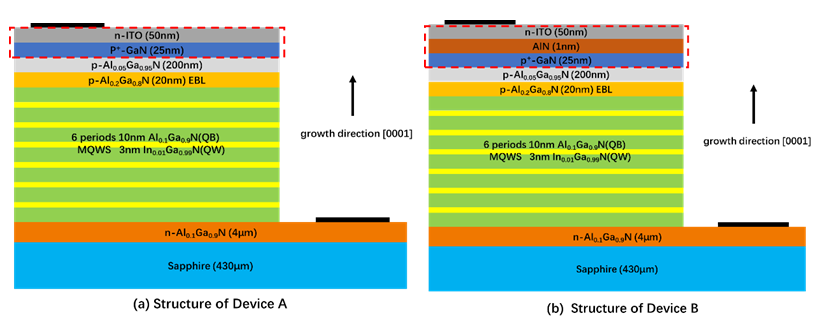Generally, a depletion region formed by p$^{+}$-GaN and ITO layers has a negative effect on hole injection, and since the concentration of holes is relatively low, the width of the depletion region is difficult to shorten (20). In this work, we proposed a structure that enhances tunneling by inserting 1nm AlN on p$^{+}$-GaN and ITO current spreading layers. When the UV LED device is applied with a forward voltage, electrons will accumulate at the interface of p$^{+}$-GaN and AlN, which narrows the width of the tunneling region. In addition, since the dielectric constant of AlN is relatively small compared to GaN, the AlN layer will produce a stronger electric field, which increases the drift velocity of the holes (21). As a result, the hole injection efficiency and light output power of the tunneling enhanced UV-LED device are improved.

## II. STRUCTURES AND PARAMETERS

As shown in Fig. 1, the UV-LED structures are arranged along  on the c-plane sapphire substrate, and the bottom layer is 4 µm Si-doped Al$_{0.1}$Ga$_{0.9}$N. Then there are the multiple quantum wells (MQWs), which includes six 3-nm In$_{0.01}$Ga$_{0.99}$N quantum wells and seven 10-nm Al$_{0.1}$Ga$_{0.9}$N quantum barriers. On the MQWs, there is a layer of 20 nm p-Al$_{0.2}$Ga$_{0.8}$N electron blocking-layer (EBL), a layer of 200 nm p-Al$_{0.05}$Ga$_{0.95}$N and 25 nm p$^{+}$-GaN ohmic contact layer. The Mg doping concentration of p$^{+}$-GaN is 10$^{20}$ cm$^{-3}$, and the hole concentration is 3×10$^{19}$ cm$^{-3}$. Compared with device A, device B only has an extra layer of 1nm AlN above the p$^{+}$-GaN layer. The size of the UV-LED chip is 300 μm × 254 μm.

Fig. 2. Numerically calculated energy band diagrams of (a) Device A, (b) Device B at 120 mA.Fig. 3. (a) Ga polarity charge distribution caused by spontaneous polarization and piezoelectric polarization of GaN and AlN, (b) the electric field distribution of device A and device B obtained by numerical calculation at a current of 120 mA.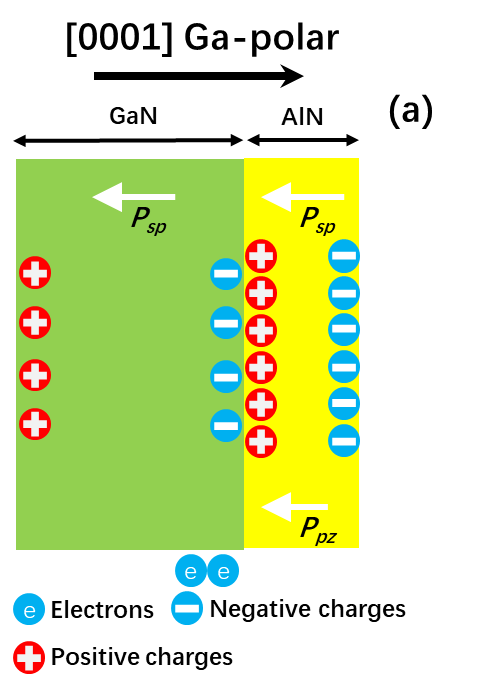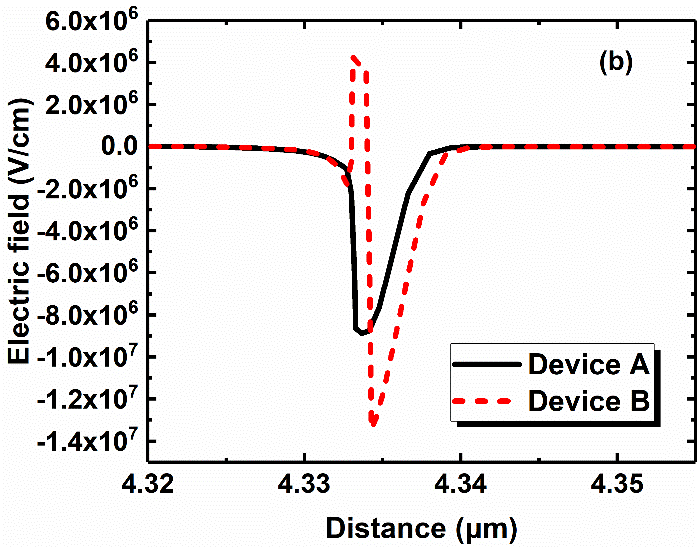The energy band diagram, hole concentration distribution, and electric field distribution of UV-LEDs are numerically investigated by the advanced semiconductor device physical model simulation software APSYS. This software can self-consistently solve Schrodinger Poisson equations, current continuity equations, quantum wave equations under suitable boundary conditions. The operating temperature, theoretical polarization charge, and Shockley-Read-Hall (SRH) recombination life are set to 300 K, 50%, and 20ns, respectively (22,23). The band offset ratio is set to 50:50 for Al$_{\mathrm x}$Ga$_{1-\mathrm x}$N / GaN and Al$_ \mathrm x$Ga$_{1- \mathrm x}$N / In$_ {\mathrm x}$Ga$_{1-\mathrm x}$N heterojunctions (24).

## III. RESULTS AND DISCUSSION

The numerically calculated energy band diagrams of Device A and Device B at 120 mA are shown in Fig. 2. As illustrated in Fig. 2(b), since the Device B forms a heterojunction with p$^{+}$-GaN and AlN, electrons accumulate at the interface between p$^{+}$-GaN and AlN, and the conduction band at the interface is pulled down, thereby shortening the width of the tunneling region.

Ga polarity charge distribution caused by spontaneous polarization and piezoelectric polarization of GaN and AlN, and the electric field distribution of device A and device B are shown in Fig. 3(a) and (b). As illustrated in Fig. 3(a), P$_{sp}$ and P$_{pz}$ represent spontaneous polarization and piezoelectric polarization, respectively. The GaN layer is completely relaxed, only P$_{sp}$ exists, but the AlN layer P$_{sp}$ and P$_{pz}$ exist (25). For the Ga-polar structure, a net positive charge exists at the GaN / AlN interface, which can attract many electrons to further accumulate at the GaN / AlN interface, thereby further shortening the width of the tunneling region, resulting in a significant increase in the probability of tunneling. Under forward bias, electrons in the p$^{+}$-GaN layer tunnel through the depletion region, leaving holes injected into the MQWs active region. Therefore, the increase in tunneling probability will increase the concentration of holes injected into the active region of MQWs, thereby increasing the efficiency of hole injection.

The electric field distributions of Devices A and B are shown in Fig. 3(b). The dielectric constants of GaN and AlN are 9.5 and 8.5 (21), respectively, and according to formula (1), a lower dielectric constant causes a higher electric field. Compared to Device A, the increase in the electric field of Device B causes higher tunneling efficiency of the carriers. As we all know, the relation between hole drift velocity ($V_{p}$) and kinetic energy ($E_{k}$) and electric field (E) is determined by the following formula (26):

##### (1)
$V_{p}=\mu_{p} E$

##### (2)
$E_{k}=0.5\mathrm{m}_{h}^{*}V_{p}^{2}$

Where $μ_{p}$ is the hole mobility, E is the electric field strength, $V_{p}$ is the drift velocity of the hole, and ${m_h}^*$ is the effective mass of the hole. The direction of the electric field is along [000-1], which is consistent with the drift direction of the holes. Since Device B has polarized charges at the interface between GaN and AlN, there are two additional electric fields in Device B, where the forward electric field has a negative effect on the hole transport and the negative electric field plays a positive role. To consider the effect of the electric field on hole injection, we integrated the electric field. The average of the integration results is -2778 mV and -3085 mV for Device A and Device B. Therefore, the holes of Device B will achieve higher drift speed and higher kinetic energy, and the hole injection efficiency is further increased.

Fig. 4. (a) Numerically calculated hole concentration distributions among MQWs active region at 120 mA, (b) current-voltage characteristics for Device A and Device B.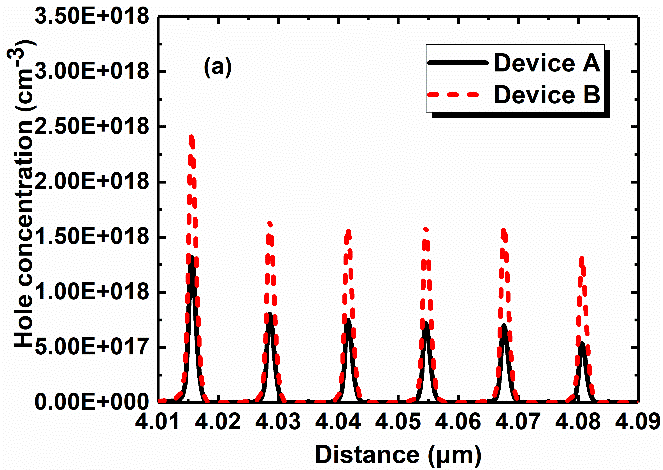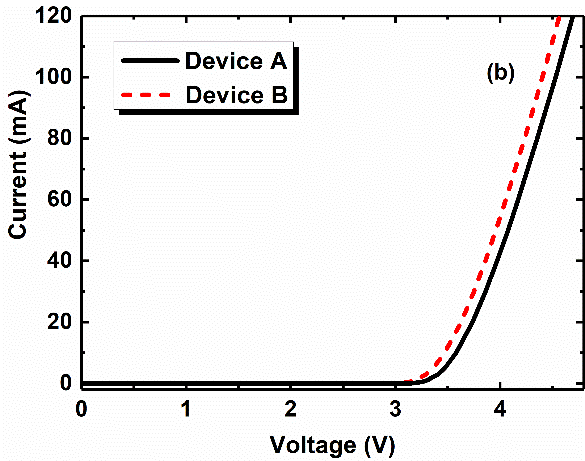The numerically calculated hole concentration distribution and current-voltage characteristics between the active regions of MQWs of device A and device B are shown in Fig. 4(a) and (b). As shown in Fig. 4(a), the tunneling-enhanced device B shows a higher hole concentration between the active regions of MQWs than the device A. As mentioned above, the tunnel enhanced Device B can reduce the width of the tunneling region, and the AlN layer with a lower dielectric constant can increase the electric field, resulting in an increase in the concentration and drift velocity of holes, thereby improving the hole injection efficiency of the UV-LED device.

Fig. 4(b) shows the numerically calculated current-voltage characteristics of Device A and Device B. When the injection current of the UV-LED device is 20mA, the forward voltage ($V_f$) of the Device A and Device B are 3.74 V and 3.63 V, respectively. the $V_f$ of Device B is slightly smaller than that of Device A, which is due to the shorter tunneling area of device B and a higher concentration of holes injected into the active region. At the same time, the added AlN layer increases the drift velocity of holes.

The internal quantum efficiency (IQE) and radiation recombination rate of the Devices A and B are shown in Fig. 5(a) and (b). To better observe the difference in the radiation recombination rate of Device A and Device B, we shifted the abscissa of Device B to the right by 0.002 μm in the figure. As illustrated in Fig. 5(a), At a current of 120 mA, the internal quantum efficiency (IQE) of device A is 38.3%, the internal quantum efficiency (IQE) of device B is 45.8%, and the IQE of device B is increased by 19.6% compared with device A.

Fig. 6. (a) EL spectrum, (b) optical output power of two devices at different injection current levels. The size for the UV-LED chips is 300 μm x 254 μm.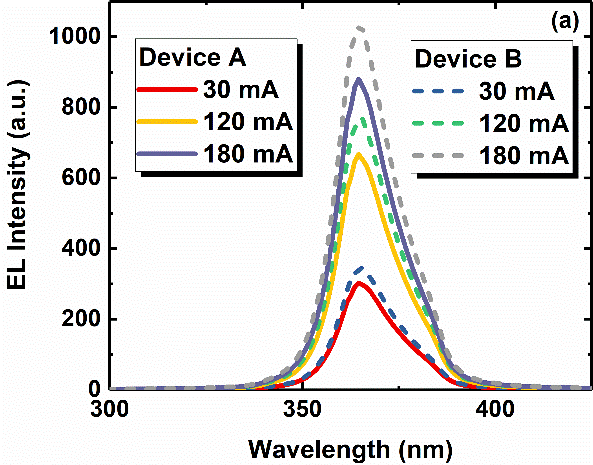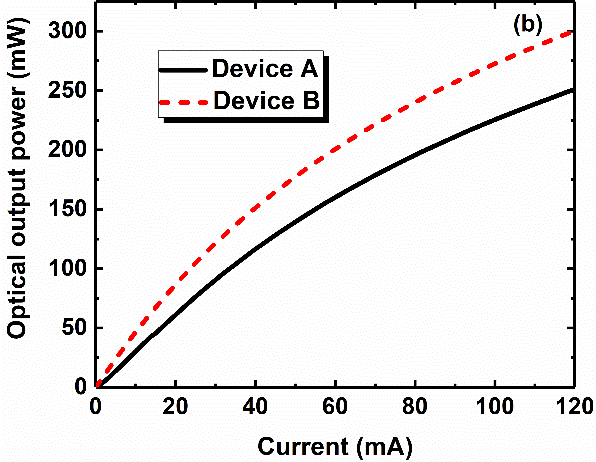From the radiative recombination rate diagram of the active region at 120 mA in Fig. 5(b), Device B exhibits higher radiation recombination efficiency, suggesting Device B has higher carrier injection efficiency compared to Device A.

As shown in Fig. 6(a) and (b), the light output power (LOP) and electroluminescence (EL) curves prove that the tunneling enhanced structure is reasonable for improving the performance of the UV-LED. Fig. 6(a) shows the electroluminescence (EL) spectra of the two devices at different currents (30 mA, 120 mA, and 180 mA, respectively). The peak emission wavelength of the two devices is approximately 365 nm. When the injection current is 30 mA, 120 mA and 180 mA, the EL intensity of Device B is always stronger than Device A.

The optical output power (LOP) of equipment A and B is shown in Fig. 6(b). When the current is 120 mA, the optical output power of device A and device B are 251 mW and 300.2 mW, respectively. Compared with device A, the LOP of device B has increased by 19.6%, which corresponds to the analysis in Fig. 2-5. The increase in optical output power of device B with enhanced tunneling is attributed to the increase in hole injection efficiency.

## V. CONCLUSIONS

In this article, we report a UV-LED structure with enhanced tunneling. Studies have shown that in the tunneling-enhanced UV-LED, the width of the tunneling region can be shortened due to the accumulation of electrons at the interface of p$^{+}$-GaN and AlN. Since the dielectric constant of the inserted AlN layer is small, the electric field strength in the tunneling region is enhanced, which further improves the hole injection efficiency. Therefore, compared with device A, the optical output power of device B at 120 mA has increased by 19.6%. The tunneling-enhanced structure provides a low-cost and simple method for increasing the hole injection efficiency and luminous efficiency of UV-LEDs

### ACKNOWLEDGMENTS

This research was funded by the National Key R&D Program of China (Grant Nos. 2016YFB0400800), the National Natural Science Foundation of China (Grant No. 61634005), and the Key R&D projects of Shaanxi Province (Grant No. 2018ZDCXL-GY-01-07).

### REFERENCES

1
Cheong H.-S., 2006, Growth and Characteristics of Near-UV LED Structures on Wet-Etched Patterned Sapphire Substrate, J. Semicond. Technol. Sci., Vol. 6, No. 3, pp. 199-2052
Kim S.Y., 2005, Enhanced Hole Injections in Organic Light Emitting Diode using Rhodium Oxide Coated Anode, J. Semicond. Technol. Sci., Vol. 5, No. 2, pp. 77-823
Khan A., 2008, Ultraviolet light-emitting diodes based on group three nitrides, Nat. Photonics, Vol. 2, No. 2, pp. 77-844
Hirayama H., 2015, Recent Progress in AlGaN‐Based Deep‐UV LEDs, Electron Commun Jpn, Vol. 98, No. 5, pp. 1-85
Tao H., 2019, Numerical Investigation on the Enhanced Performance of N-Polar AlGaN-Based Ultraviolet Light-Emitting Diodes With Superlattice p-Type Doping, IEEE Trans. Electron Dev, Vol. 66, No. 1, pp. 478-4846
Wang Y., 2020, Using a Multi-Layer Stacked AlGaN/GaN Structure to Improve the Current Spreading Performance of Ultraviolet Light-Emitting Diodes, Materials, Vol. 13, No. 2, pp. 4547
Verzellesi G., 2013, Efficiency droop in InGaN/GaN blue light-emitting diodes: Physical mechanisms and remedies, J. Appl. Phys., Vol. 1148
Li D., 2018, AlGaN photonics: recent advances in materials and ultraviolet devices, Adv. Opt. Photonics., Vol. 10, No. 1, pp. 43-1109
Maeda N., 2013, Realization of high-efficiency deep-UV LEDs using transparent p-AlGaN contact layer, Phys. Status Solidi C, Vol. 10, No. 11, pp. 1521-152410
Zhang J., 2001, Pulsed atomic layer epitaxy of quaternary AlInGaN layers, Appl. Phys. Letter., Vol. 79, No. 7, pp. 925-92711
Fareed R.S., 2004, High quality InN/GaN heterostructures grown by migration enhanced metalorganic chemical vapor deposition, Appl. Phys. Letter., Vol. 84, No. 11, pp. 1892-189412
Nakamura S., 1998, Present status of InGaN/GaN/AlGaN-based laser diodes, J. Cryst. Growth., Vol. 189, pp. 820-82513
Knauer A., 2013, AlGaN layer structures for deep UV emitters on laterally overgrown AlN/sapphire templates, Phys. Status Solidi A., Vol. 210, No. 3, pp. 451-45414
Dong P., 2013, 282-nm AlGaN-based deep ultraviolet light-emitting diodes with improved performance on nano-patterned sapphire substrates, Appl. Phys. Lett., Vol. 102, No. 24, pp. 24111315
Fan X.C., 2015, Efficiency improvements in AlGaN-based deep ultraviolet light-emitting diodes using inverted-V-shaped graded Al composition electron blocking layer, Superlattice Microst., Vol. 88, No. 88, pp. 467-47316
Simon J., 2010, Polarization-Induced Hole Doping in Wide-Band-Gap Uniaxial Semiconductor Heterostructures, Science, Vol. 327, No. 5961, pp. 60-6417
Sun P., 2015, Advantages of AlGaN-based deep ultraviolet light-emitting diodes with a superlattice electron blocking layer, Superlattice Microst., Vol. 85, pp. 59-6618
Zhang Z.-H., 2016, On the hole accelerator for III-nitride light-emitting diodes, Appl. Phys. Lett., Vol. 108, No. 15, pp. 15110519
Zhang Z.-H., 2013, InGaN/GaN light-emitting diode with a polarization tunnel junction, Appl. Phys. Lett., Vol. 102, No. 19, pp. 19350820
Lin C.F., 2000, Properties of Mg activation in thermally treated GaN: Mg films, J. Appl. Phys., Vol. 88, No. 11, pp. 6515-651821
Li L.P., 2017, A dielectric-constant-controlled tunnel junction for III-nitride light-emitting diodes, Phys. Status Solidi A, Vol. 214, No. 6, pp. 160093722
Bernardini F., 1997, Spontaneous polarization and piezoelectric constants of III-V nitrides, Phys. Rev. B., Vol. 56, No. 16, pp. 10024-1002723
Fiorentini V., 2002, Evidence for nonlinear macroscopic polarization in III-V nitride alloy heterostructures, Appl. Phys. Lett., Vol. 80, No. 7, pp. 1204-120624
Piprek J., 2010, Efficiency droop in nitride-based light-emitting diodes, Phys. Status Solidi A, Vol. 207, No. 10, pp. 2217-222525
Ambacher O., 2000, Two dimensional electron gases induced by spontaneous and piezoelectric polarization in undoped and doped AlGaN/GaN heterostructures, J. Appl. Phys., Vol. 87, No. 1, pp. 334-34426
Lang J., 2019, High performance of AlGaN deep-ultraviolet light emitting diodes due to improved vertical carrier transport by delta-accelerating quantum barriers, Appl. Phys. Lett., Vol. 114, No. 17, pp. 172105## Author

Jinxing Wu was born in Tongchuan City, Shaanxi Province, China in 1993.

Received a master's degree from Xidian University in 2018.

He is currently studying for a Ph.D. in Wide Bandgap Semiconductor Tech-nology Disciplines State Key Laboratory, School of Advanced Materials and Nanotechnology, Xidian University.

His interests include AlGaN material epitaxy and high-efficiency AlGaN-based UV-LED devices.

Peixian Li was born in 1972, is a professor and doctoral supervisor at Xidian University.

Young science and technology star of Shaanxi Province, China, innovative and entrepreneurial talents in Shaanxi Province, and second-class expert of Shaanxi Provincial SASAC.

Head of Optoelectronics Research at the State Key Subject Laboratory of Wide Band Gap Semiconductor Materials and Devices.

Affiliated to the National Innovation Team of Academician of Yue Hao Academy of Sciences.

Engaged in the research of GaN-based optoelectronic materials and devices.

Hosted or participated in more than 10 projects above the provincial and ministerial level and obtained a large number of scientific research results with industrial practical value.

Xiaowei Zhou is an associate professor of Xidian University.

Affiliated to the National Innovation Team of Academician of Yue Hao Academy of Sciences.

State Key Subject Laboratory of Wide Band Gap Semiconductor Materials and Devices.

The main research directions are: nitride semiconductor material growth technology; GaN-based deep ultraviolet LED materials and devices; new GaN-based heterojunction material technology.